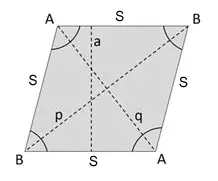# Rhombus Calculator

Our Rhombus Calculator is a handy tool that can help you find all the mathematical values of a rhombus, including its area, perimeter, diagonals, and angles.

*Please note that changing one of the dimensions may alter the values of other dimensions.

## What is a Rhombus?

A rhombus can be defined as a quadrilateral that has four sides that are equal to each other in length. It also looks similar to a diamond or a kite.

The opposite sides are parallel and opposite angles are equal. The two diagonals bisect each other at right angles.

Another important feature of a rhombus is that its opposite sides are parallel to each other and its opposite angles are equal. The two diagonals of a rhombus bisect each other at right angles. A rhombus can also be referred to as a parallelogram.

Rhombus Shape Calculator°

°

You may set the number of decimal places in the online calculator. By default there are only two decimal places.

Results
Area: 0.00 sq. units
Perimeter: 0.00 units

### The following algorithms are the functions that the calculator is capable of carrying out:

Angle A = 180 - Angle B

Angle B = 180 - Angle A

Altitude = sin(B) x S (Sine of Angle B multiplied by Side length)

Area can be calculated several ways:

Area = a x S (Altitude x Sides)

Area = S2 x sin(A) (Side length squared multiplied by the sine of Angle A)

Area = S2 x sin(B) (Side length squared multiplied by the sine of Angle B)

Area = (p x q)/2 (diagonal A-A (p) multiplied by diagonal B-B (q) then the result is divided by two)

Perimeter = 4 x S (Side length multiplied by 4)